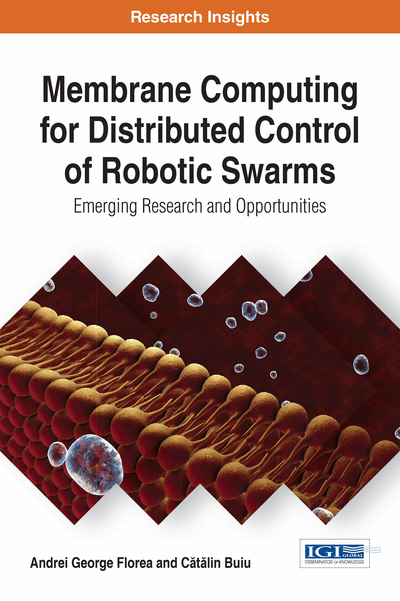# Membrane Computing: Theory and Applications

DOI: 10.4018/978-1-5225-2280-5.ch002
Available
\$37.50
No Current Special Offers

## Abstract

The theoretical computing models that are used throughout this book are described in this chapter. These models are based on the initial P system model and include: Numerical P systems, Enzymatic Numerical P systems, P colonies and P swarms. Detailed examples and execution diagrams help the reader allow the reader to understand the functioning principle of each model and also its potential in various applications. The similarity between P systems (and their variants) and robot control models is also addressed. This analysis is presented to the reader in a side-by-side manner using a table where each row represents an analysis topic. Among others we mention: (1) Architectural structure, (2) Modularity and hierarchy, (3) Input-output relationships, (4) Parallelism.
Chapter Preview
Top

## Introduction

P systems were introduced in a technical report from 1998 by the Romanian mathematician Gheorghe Păun and are at the foundation of membrane computing. The purpose of this chapter is to give an overview of the basic principles and models in membrane computing. Starting from analogies with biomembranes, the structure of a standard P system is presented. Then, several variants of the original model are discussed. The main paradigms that are relevant from the point of view of robotics applications, including standard numerical P systems, enzymatic numerical P systems, P colonies, and P swarms, are presented with examples.

Membrane computing is part of the larger field of molecular computing. DNA computing is perhaps the most known paradigm of molecular computing (Amos, Păun, Rozenberg, & Salomaa, 2002) and was initiated by the early paper of Adleman in which he described the use of DNA as a computational system (Adleman, 1994) and also implemented a relevant computation in a biological laboratory.

The models of molecular computation are categorized in (Amos, 2010) in four categories: filtering, splicing, constructive, and membrane-based

Filtering models are based on computations performed on finite multisets of strings. The concept of a multiset will be detailed in the discussion on P systems. For more details about filtering models, the interested reader is referred to (Amos, 2010). Splicing systems have been introduced in the seminal paper of Tom Head from 1987 (Head, 1987). The splice operation, given two strings S and T, is identical to the crossover operator employed by genetic algorithms (Holland, 1992). A restricted form of the splicing operation is used in the Parallel Associative Memory Model introduced in (Reif & H., 1995). Constructive models are based on the principles of self-assembly, and an example is the Tile Assembly Model introduced in (Rothemund & Winfree, 2000).

The most well-known class of membrane models is that of P systems, introduced by the Romanian mathematician Gheorghe Păun (Păun, 2000) and this will be described in the following part of this chapter. Next we will give a detailed analogy between P systems and swarm robotic systems that lays the foundations for our original contributions presented in Chapter 4.

## Complete Chapter List

Search this Book:
Reset